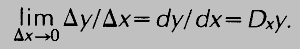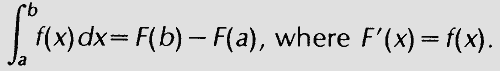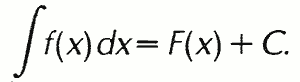# calculus

(redirected from urate calculus)
Also found in: Dictionary, Thesaurus, Medical.

## calculus,

branch of mathematicsmathematics,
deductive study of numbers, geometry, and various abstract constructs, or structures; the latter often "abstract" the features common to several models derived from the empirical, or applied, sciences, although many emerge from purely mathematical or logical
that studies continuously changing quantities. The calculus is characterized by the use of infinite processes, involving passage to a limitlimit,
in mathematics, value approached by a sequence or a function as the index or independent variable approaches some value, possibly infinity. For example, the terms of the sequence 1-2, 1-4, 1-8, 1-16, … are obviously getting smaller and smaller; since, if enough
—the notion of tending toward, or approaching, an ultimate value. The English physicist Isaac NewtonNewton, Sir Isaac,
1642–1727, English mathematician and natural philosopher (physicist), who is considered by many the greatest scientist that ever lived. Early Life and Work
and the German mathematician G. W. LeibnizLeibniz or Leibnitz, Gottfried Wilhelm, Baron von
, 1646–1716, German philosopher and mathematician, b. Leipzig.
, working independently, developed the calculus during the 17th cent. The calculus and its basic tools of differentiation and integration serve as the foundation for the larger branch of mathematics known as analysisanalysis,
branch of mathematics that utilizes the concepts and methods of the calculus. It includes not only basic calculus, but also advanced calculus, in which such underlying concepts as that of a limit are subjected to rigorous examination; differential and integral
. The methods of calculus are essential to modern physics and to most other branches of modern science and engineering.

### The Differential Calculus

The differential calculus arises from the study of the limit of a quotient, Δyx, as the denominator Δx approaches zero, where x and y are variables. y may be expressed as some functionfunction,
in mathematics, a relation f that assigns to each member x of some set X a corresponding member y of some set Y; y is said to be a function of x, usually denoted f(x) (read "f of x ").
of x, or f(x), and Δy and Δx represent corresponding increments, or changes, in y and x. The limit of Δyx is called the derivative of y with respect to x and is indicated by dy/dx or Dxy:The symbols dy and dx are called differentials (they are single symbols, not products), and the process of finding the derivative of y=f(x) is called differentiation. The derivative dy/dx=df(x)/dx is also denoted by y′, or f′(x). The derivative f′(x) is itself a function of x and may be differentiated, the result being termed the second derivative of y with respect to x and denoted by y″, f″(x), or d2y/dx2. This process can be continued to yield a third derivative, a fourth derivative, and so on. In practice formulas have been developed for finding the derivatives of all commonly encountered functions. For example, if y=xn, then y′=nxn − 1, and if y=sin x, then y′=cos x (see trigonometrytrigonometry
[Gr.,=measurement of triangles], a specialized area of geometry concerned with the properties of and relations among the parts of a triangle. Spherical trigonometry is concerned with the study of triangles on the surface of a sphere rather than in the plane; it is
). In general, the derivative of y with respect to x expresses the rate of change in y for a change in x. In physical applications the independent variable (here x) is frequently time; e.g., if s=f(t) expresses the relationship between distance traveled, s, and time elapsed, t, then s′=f′(t) represents the rate of change of distance with time, i.e., the speed, or velocity.

Everyday calculations of velocity usually divide the distance traveled by the total time elapsed, yielding the average velocity. The derivative f′(t)=ds/dt, however, gives the velocity for any particular value of t, i.e., the instantaneous velocity. Geometrically, the derivative is interpreted as the slope of the line tangent to a curve at a point. If y=f(x) is a real-valued function of a real variable, the ratio Δyx=(y2y1)/(x2x1) represents the slope of a straight line through the two points P (x1,y1) and Q (x2,y2) on the graph of the function. If P is taken closer to Q, then x1 will approach x2 and Δx will approach zero. In the limit where Δx approaches zero, the ratio becomes the derivative dy/dx=f′(x) and represents the slope of a line that touches the curve at the single point Q, i.e., the tangent line. This property of the derivative yields many applications for the calculus, e.g., in the design of optical mirrors and lenses and the determination of projectile paths.

### The Integral Calculus

The second important kind of limit encountered in the calculus is the limit of a sum of elements when the number of such elements increases without bound while the size of the elements diminishes. For example, consider the problem of determining the area under a given curve y=f(x) between two values of x, say a and b. Let the interval between a and b be divided into n subintervals, from a=x0 through x1, x2, x3, … xi − 1, xi, … , up to xn=b. The width of a given subinterval is equal to the difference between the adjacent values of x, or Δxi=xixi − 1, where i designates the typical, or ith, subinterval. On each Δxi a rectangle can be formed of width Δxi, height yi=f(xi) (the value of the function corresponding to the value of x on the right-hand side of the subinterval), and area ΔAi=f(xixi. In some cases, the rectangle may extend above the curve, while in other cases it may fail to include some of the area under the curve; however, if the areas of all these rectangles are added together, the sum will be an approximation of the area under the curve.

This approximation can be improved by increasing n, the number of subintervals, thus decreasing the widths of the Δx's and the amounts by which the ΔA's exceed or fall short of the actual area under the curve. In the limit where n approaches infinity (and the largest Δx approaches zero), the sum is equal to the area under the curve:The last expression on the right is called the integral of f(x), and f(x) itself is called the integrand. This method of finding the limit of a sum can be used to determine the lengths of curves, the areas bounded by curves, and the volumes of solids bounded by curved surfaces, and to solve other similar problems.

An entirely different consideration of the problem of finding the area under a curve leads to a means of evaluating the integral. It can be shown that if F(x) is a function whose derivative is f(x), then the area under the graph of y=f(x) between a and b is equal to F(b) − F(a). This connection between the integral and the derivative is known as the Fundamental Theorem of the Calculus. Stated in symbols:The function F(x), which is equal to the integral of f(x), is sometimes called an antiderivative of f(x), while the process of finding F(x) from f(x) is called integration or antidifferentiation. The branch of calculus concerned with both the integral as the limit of a sum and the integral as the antiderivative of a function is known as the integral calculus. The type of integral just discussed, in which the limits of integration, a and b, are specified, is called a definite integral. If no limits are specified, the expression is an indefinite integral. In such a case, the function F(x) resulting from integration is determined only to within the addition of an arbitrary constant C, since in computing the derivative any constant terms having derivatives equal to zero are lost; the expression for the indefinite integral of f(x) isThe value of the constant C must be determined from various boundary conditions surrounding the particular problem in which the integral occurs. The calculus has been developed to treat not only functions of a single variable, e.g., x or t, but also functions of several variables. For example, if z=f(x,y) is a function of two independent variables, x and y, then two different derivatives can be determined, one with respect to each of the independent variables. These are denoted by ∂z/∂x and ∂z/∂y or by Dxz and Dyz. Three different second derivatives are possible, ∂2z/∂x2, ∂2z/∂y2, and ∂2z/∂xy=∂2z/∂yx. Such derivatives are called partial derivatives. In any partial differentiation all independent variables other than the one being considered are treated as constants.

### Bibliography

See R. Courant and F. John, Introduction to Calculus and Analysis, Vol. I (1965); M. Kline, Calculus: An Intuitive and Physical Approach (2 vol., 1967); G. B. Thomas and R. L. Finney, Calculus and Analytic Geometry (7th ed. 2 vol., 1988).

The following article is from The Great Soviet Encyclopedia (1979). It might be outdated or ideologically biased.

## Calculus

a formal apparatus, based on clearly formulated rules, for operating with symbols of a specified type, which permits an exhaustively exact description of a certain class of problems as well as solution algorithms for certain subclasses of this class. The subclasses in question coincide with the whole class only in the case of the simplest calculi. Examples of calculi are the set of arithmetic rules for operating with numbers (that is, numerical symbols), the literal calculus of elementary algebra, differential calculus, integral calculus, the calculus of variations, and other branches of mathematical analysis and the theory of functions.

Despite its early origin, the term “calculus” was used in mathematics without a rigorous general definition until very recently. With the development of mathematical logic a demand arose for a general theory of calculus as well as for the refinement of the concept of “calculus” itself, which underwent a more systematic formalization. In most cases, however, the following conception of a calculus (originating from D. Hilbert) proves to be sufficient. Consider a certain alphabet (generally speaking, infinite, although also possibly given by means of a finite number of symbols), whose elements, called letters, are used to construct formulas of the calculus under consideration (sometimes called words or expressions) with the help of clearly stated formation rules. Some of these (“well-formed”) formulas are declared to be axioms, and from these, with the help of transformation rules (or rules of deduction), new formulas are “deduced,” which are called theorems of the given calculus. Sometimes the term “calculus” is applied only to the “dictionary” (“expression”) part of the structure described, and it is said that joining the “deductive” part to it (that is, adding both the rules and axioms of formation to the rules of deduction and to the alphabet) produces a formal system. Besides, these terms are often also considered synonymous (and the terms “logistic system,” “formal theory,” “formalism,” and many others are also used as synonyms for this). If such a noninterpreted (“meaningless”) calculus is juxtaposed to a certain interpretation (or, as is said, a purely syntactic treatment is supplemented by semantics), then a formalized language is obtained. The representation of substantive logical (and logico-mathematical) theories in the form of formalized languages is a characteristic feature of mathematical logic.

### REFERENCES

Kleene, S. C. Vvedenie v metamatematiku, sections 14–20. Moscow, 1957. (Translated from English.)
Markov, A. A. “Teoriia algorifmov.” Moscow-Leningrad, 1954. (Tr. Matematicheskogo in-ta im. V. A. Steklova, vol. 42.)
Curry, H. B. Osnovaniia matemalicheskoi logiki, chap. 2. Moscow, 1969. (Translated from English.)
Matematicheskaia teoriia logicheskogo vyvoda.Collection of translations. Edited by A. V. Idel’son and G. E. Mints. Moscow, 1967.
Logicheskie i logiko-matematicheskie ischisleniia, 1.Collection of works. Edited by V. P. Orevkov. Leningrad, 1968.

IU. A. GASTEV

## calculus

[′kal·kyə·ləs]
(anatomy)
A small and cuplike structure.
(mathematics)
The branch of mathematics dealing with differentiation and integration and related topics.
(pathology)
An abnormal, solid concretion of minerals and salts formed around organic materials and found chiefly in ducts, hollow organs, and cysts.
McGraw-Hill Dictionary of Scientific & Technical Terms, 6E, Copyright © 2003 by The McGraw-Hill Companies, Inc.

## calculus

1. a branch of mathematics, developed independently by Newton and Leibniz. Both differential calculus and integral calculus are concerned with the effect on a function of an infinitesimal change in the independent variable as it tends to zero.
2. any mathematical system of calculation involving the use of symbols
3. Logic an uninterpreted formal system
4. Pathol a stonelike concretion of minerals and salts found in ducts or hollow organs of the body
Collins Discovery Encyclopedia, 1st edition © HarperCollins Publishers 2005
Site: Follow: Share:
Open / Close